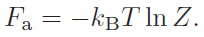## Thursday, September 2, 2010

### Week 6 (1.09.10) meeting minutes

The topic for this weeks meeting was Chapter 6: Entropy, Temperature and Free Energy from Biological Physics by Nelson.

Students were asked what they thought the most important concepts from the chapter were, the topics mentioned were:
• Gibbs Free Energy
• The Fundamental Definition of Temperature
• The Sakur-Tetrode formula for Entropy
• The example of RNA folding
• The Partition Function
• The Zeroth Law
• What is Phase Space?
Sakur-Tetrode formula for Entropy:

The Sakur-Tetrode formula gives an exact description of entropy for non-interacting particles. This is good for non-interacting particles at a large volume: as the volume is reduced the ideal conditions break down. In general this formula cannot be applied to cells due to these constraints. Essentially it is correct for an ideal gas or osmotic pressures at equilibrium at low concentrations.

Some of the terms in the full expression of the formula were queried.

Ross described the reasons for the inclusion of Planck's constant in the formula as profound. He summarized this idea by saying that in phase space there is one quantum state per volume, and this volume corresponds to Planck's constant. For simple purposes it is included to fix the units required for the formula.

The factorial term comes from the definition of entropy and accounts for the particles with identical chemical composition being indistinguishable. The exclusion of this factorial, for example using just N instead of N!, leads to Gibb's paradox. Gibb's paradox results from an incorrect form of the the entropy equations which does not lead to entropy being additive.

The Partition Function:

The partition function, Z, gives the sum over all the possible configurations for the particle with energy Ej. The example given in Chapter 6 was if you want to minimize the free energy of system a, Fa, by calculating Z you can show the Fa_min is just:It was stated that calculating Z for any interacting system is not trivial, and in fact very clever people spend careers trying to calculate Z for interacting systems. One application of this partition function in terms of free energy is calculating the free energy of a drug bound in a binding pocket of an enzyme.

Gibbs Free Energy:The terms Ea + pVa is known as the enthalpy (at fixed volume and temperature). pVa is the work done and Ea is the internal energy.

The Zeroth Law

The Zeroth Law is named as such as it was discovered after the first three laws of thermodynamics, however, it logically underpins the following three laws.

Lastly we discusses phase space.

What is Phase Space?

Instead of representing a system in three dimension of space, in phase space each particle is represented by a vector in each of 6 dimensions, one for each spatial dimension of both the particles position and momentum. An example of a representation of a Phase space diagram is a porcupine diagram of a protein.

Lastly the famous Australian scientist Dennis Evans was name dropped. He was the first to analytically prove the second law of thermodynamics, what a fantastic result.